General Information

Below are online physics problems for First Year Physics: Fluids, Gravity, Oscillations, Waves, Thermodynamics, Phy132. Pick a problem below and start by clicking on "New n1 & n2". After determining the answer, enter it in the text box "Input n3:", then click "Grade it". The program will display "correct" or "incorrect". If you want to try the calculation again click "New n1 & n2". All answers are integers. You should not need a calculator to solve the problems. Only type an integer as an answer. If my students want credit for answering the problem correctly, then type in your id and click "record grade". Let me know if you have any difficulties in understanding the questions.

## Phy132

### Fluids: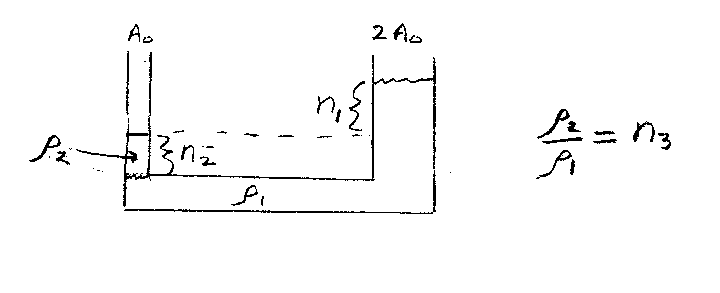Problem A1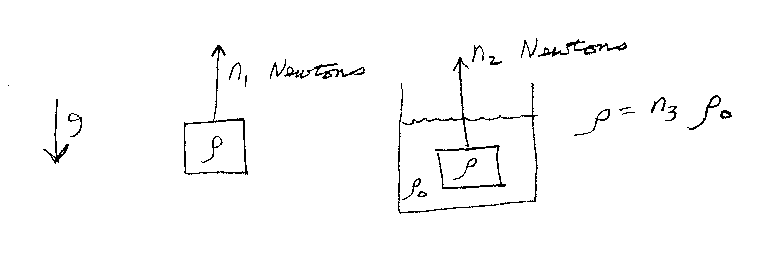Problem A2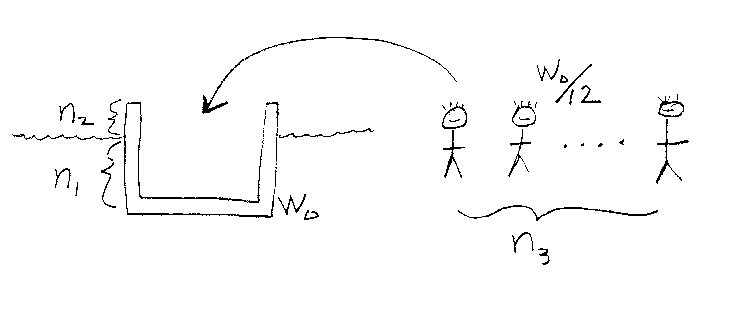Problem A3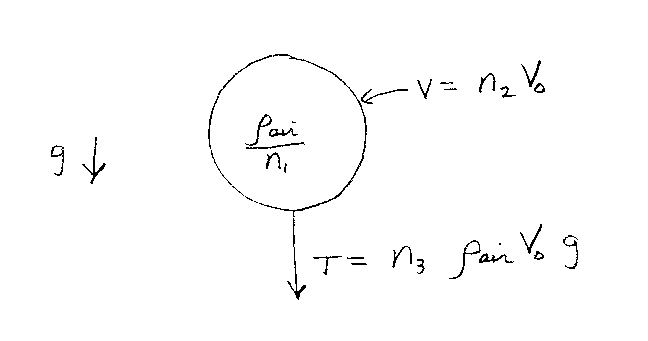Problem A4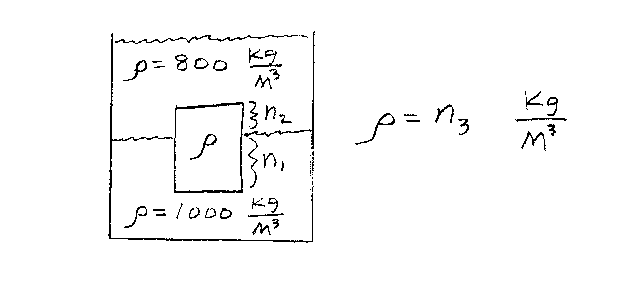Problem A5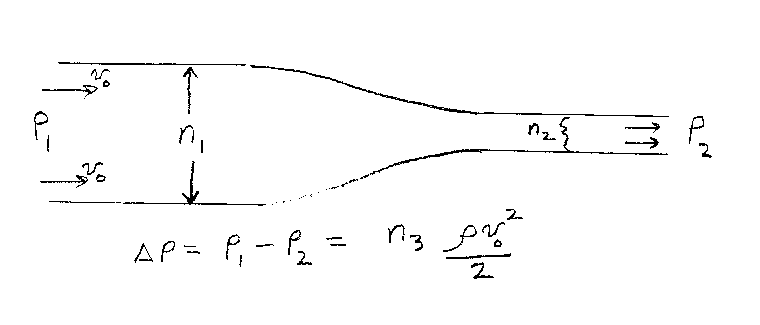Problem A6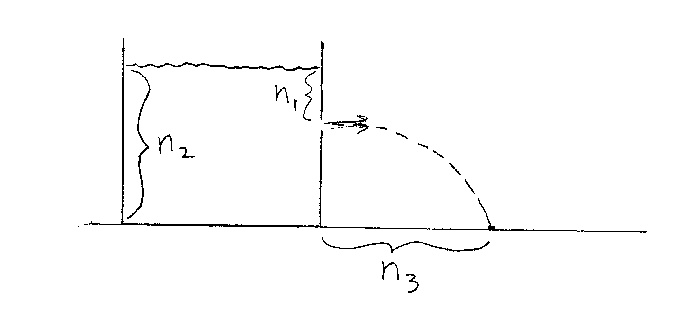Problem A7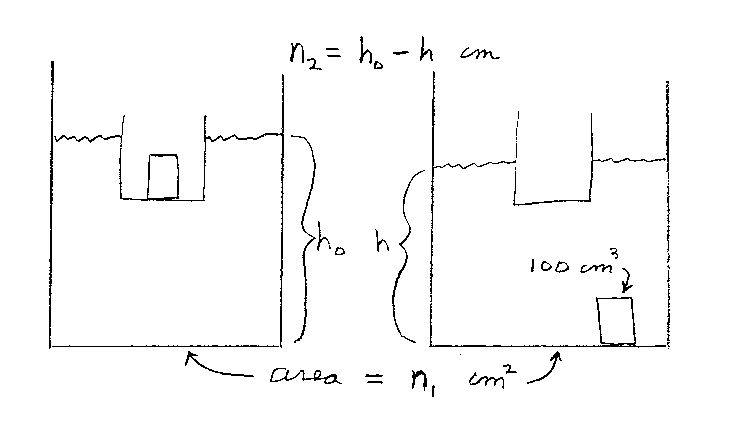Problem A8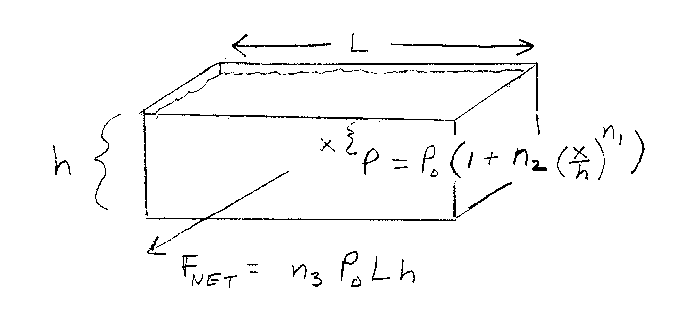Problem A9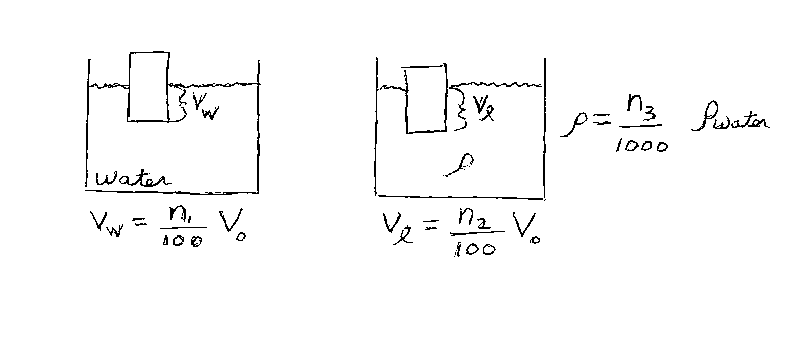Problem A10

### Newtonian Gravitation: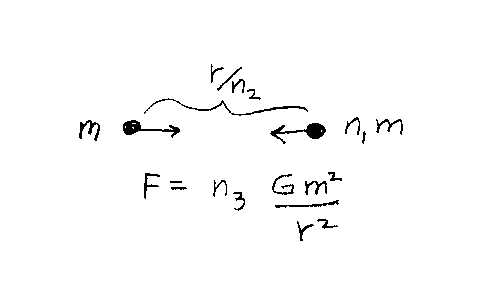Problem B1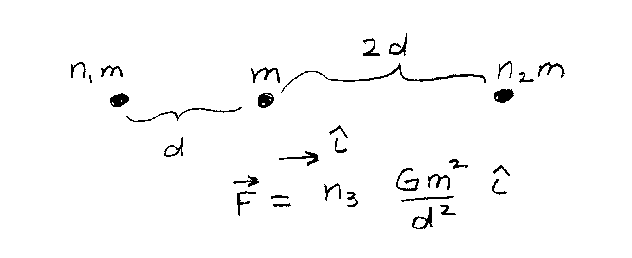Problem B2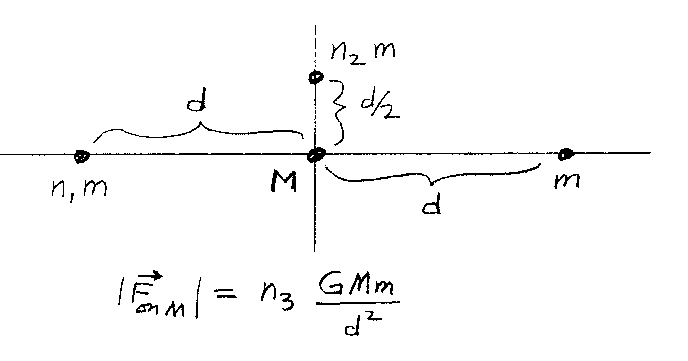Problem B3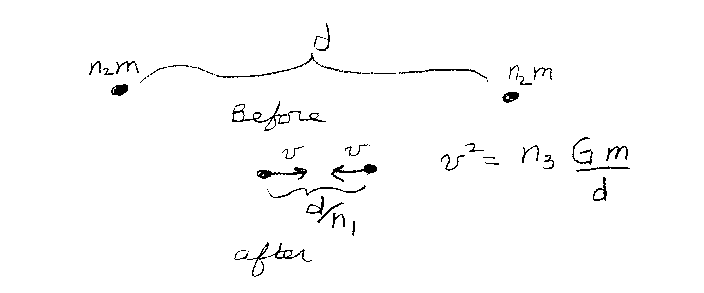Problem B4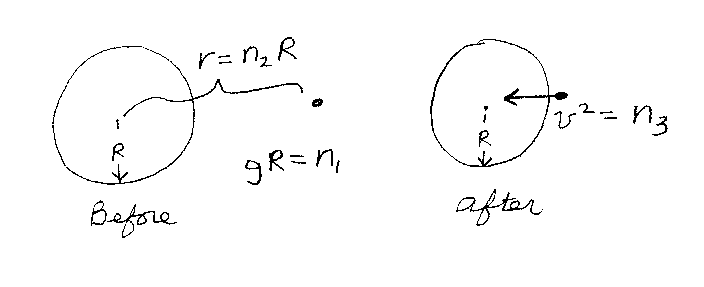Problem B5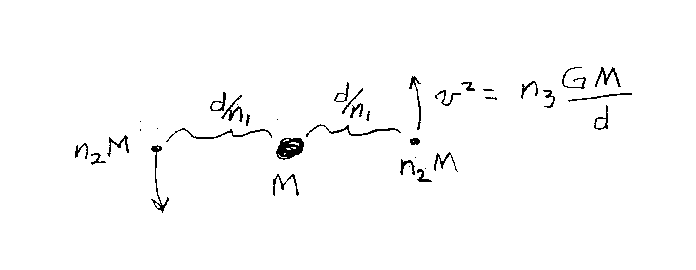Problem B6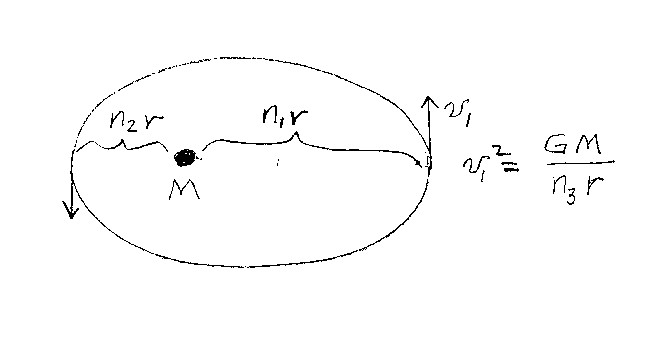Problem B7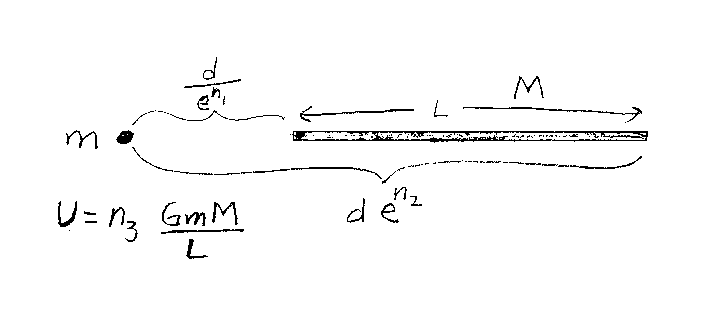Problem B8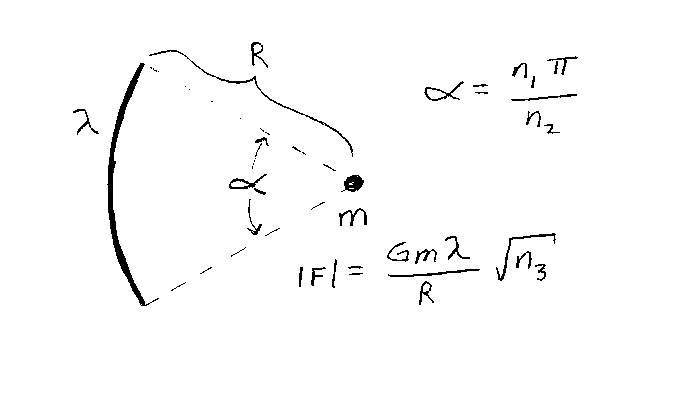Problem B9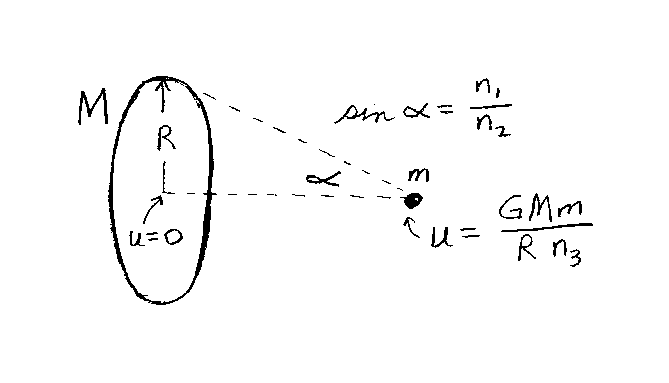Problem B10

### Oscillations and Waves: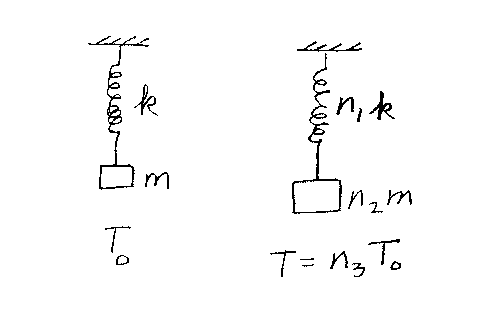Problem C1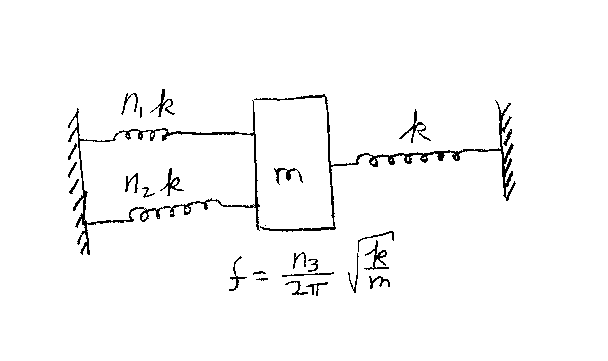Problem C2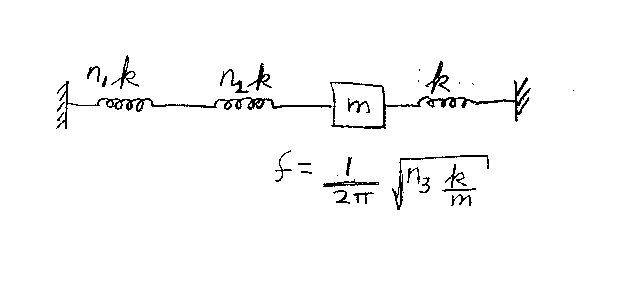Problem C3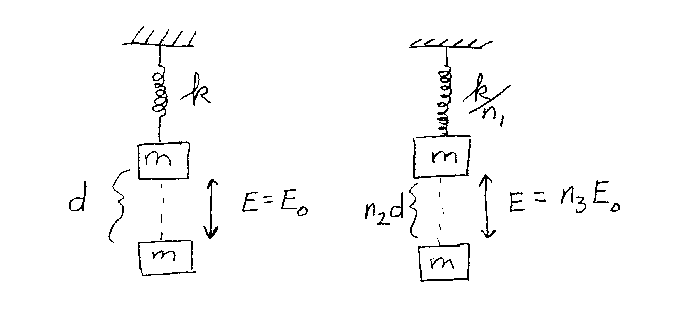Problem C4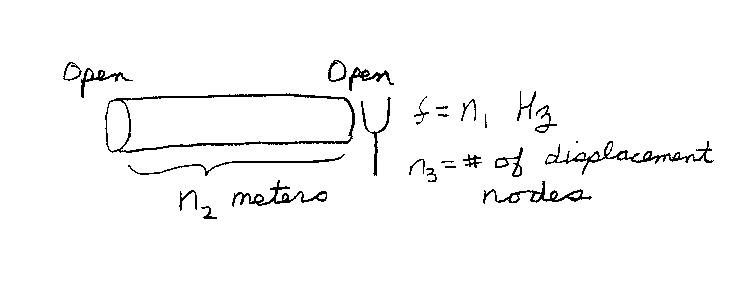Problem C5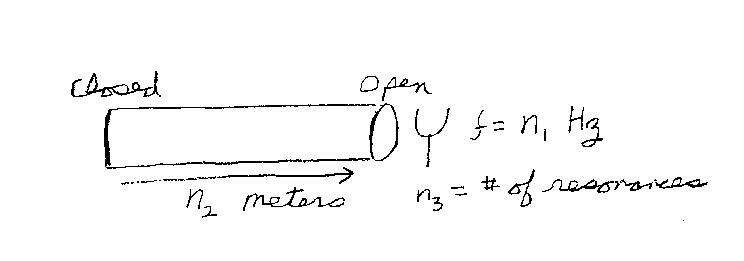Problem C6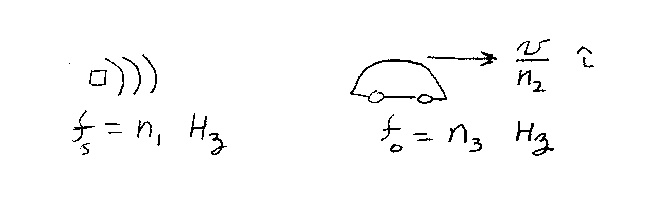Problem C7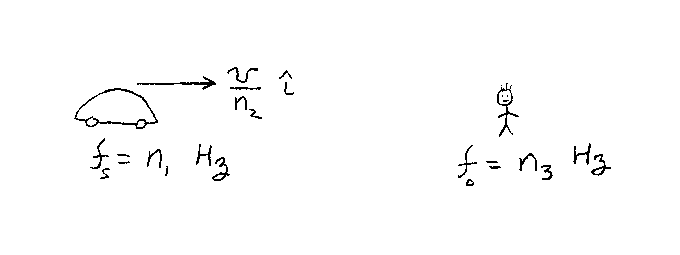Problem C8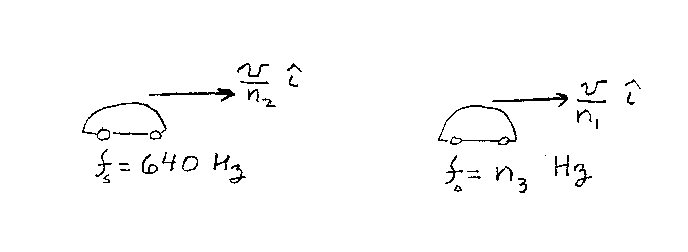Problem C9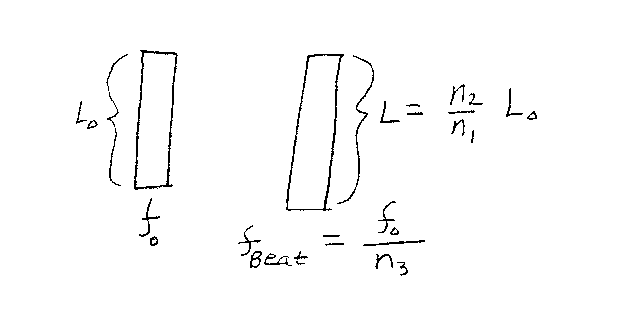Problem C10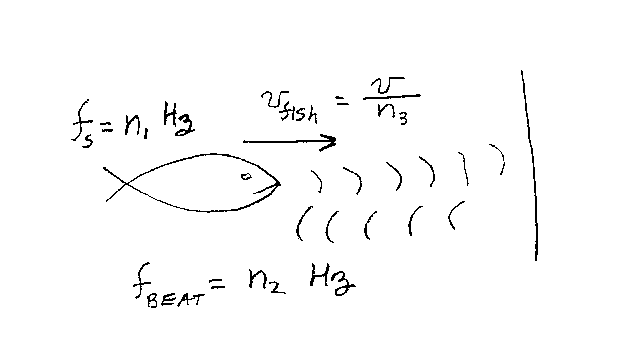Problem C11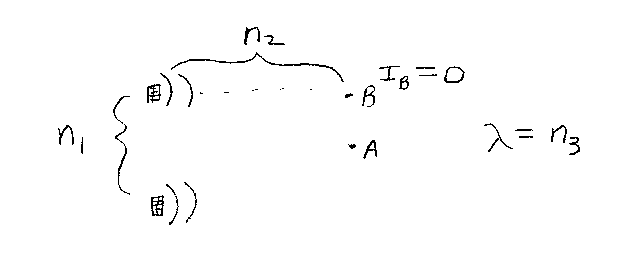Problem C12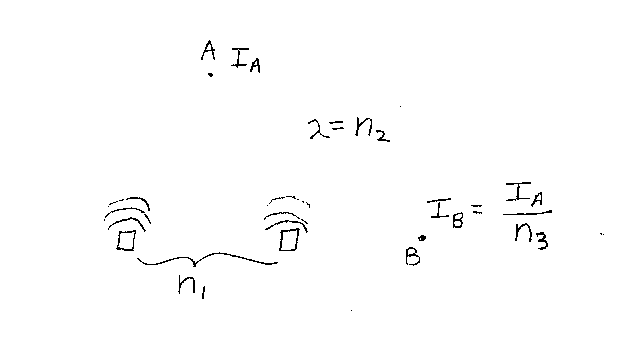Problem C13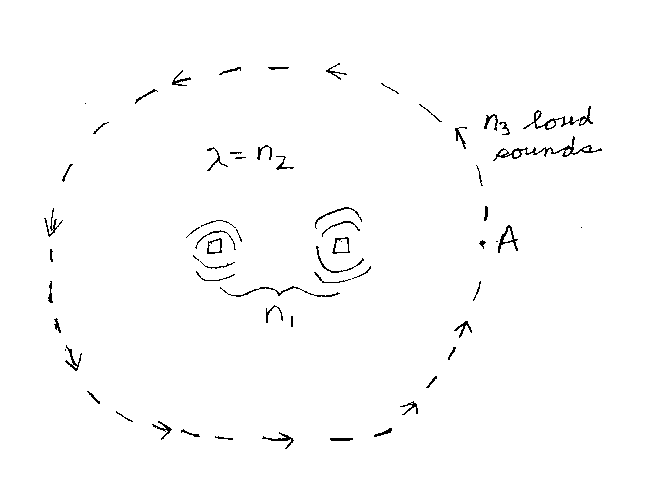Problem C14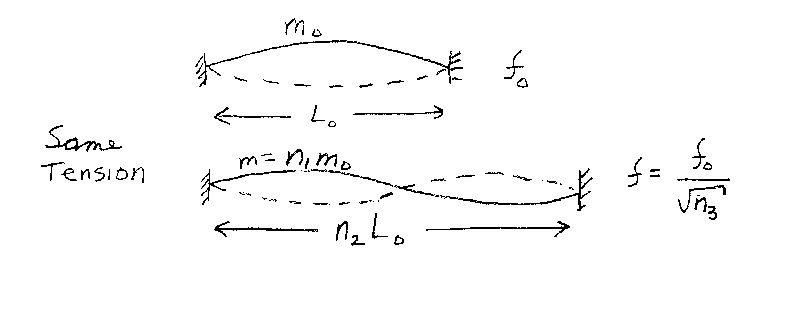Problem C15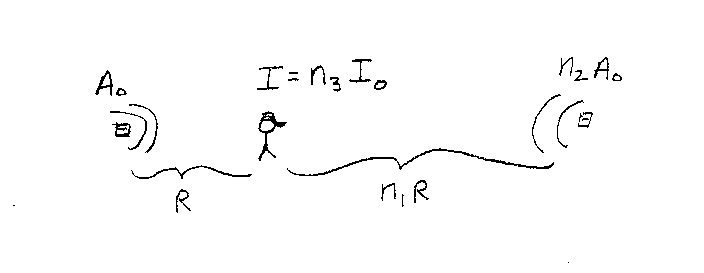Problem C16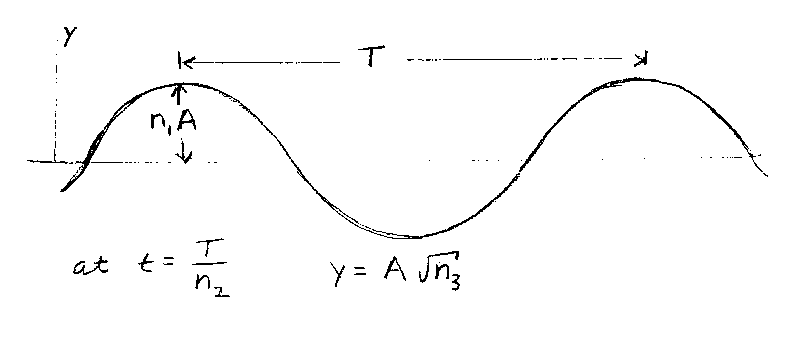Problem C17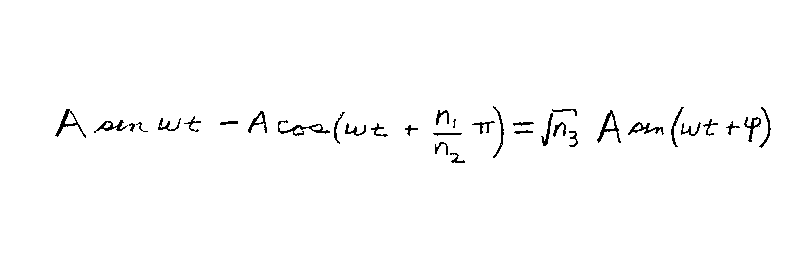Problem C18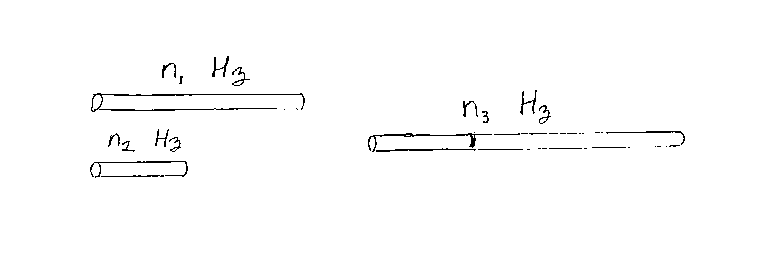Problem C19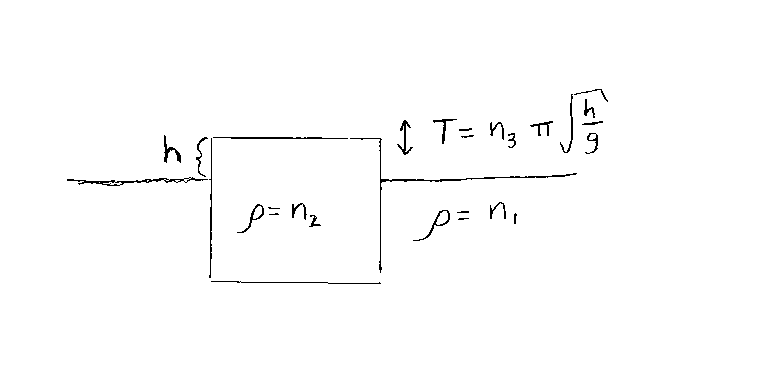Problem C20

### Thermodynamics: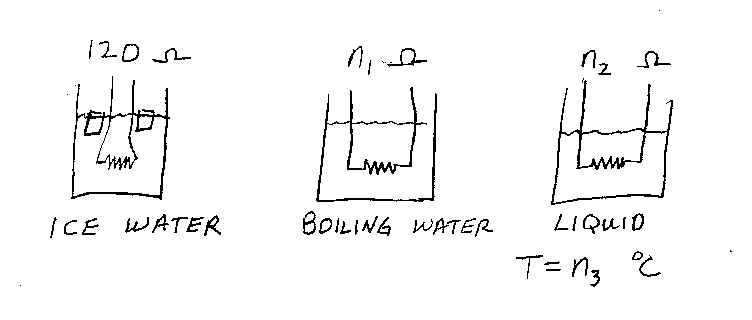Problem D1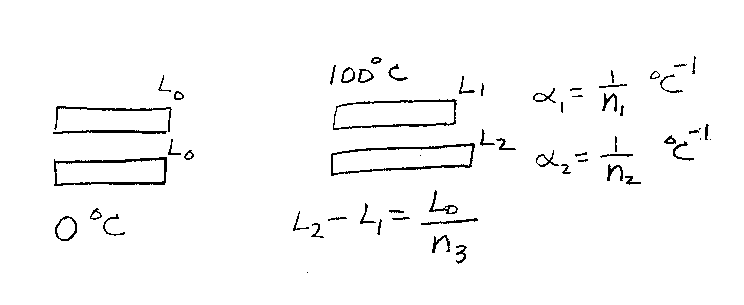Problem D2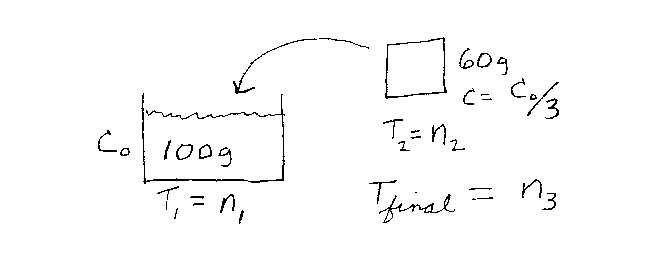Problem D3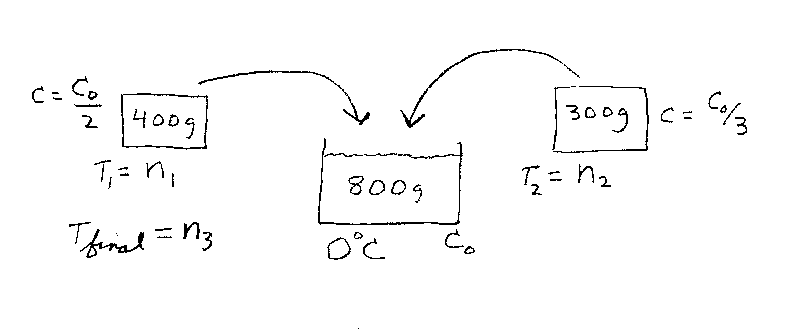Problem D4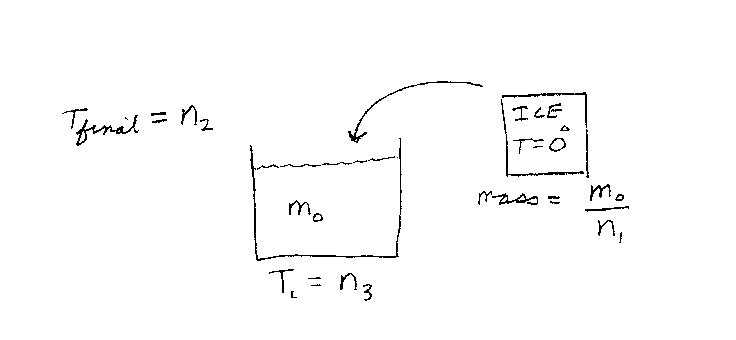Problem D5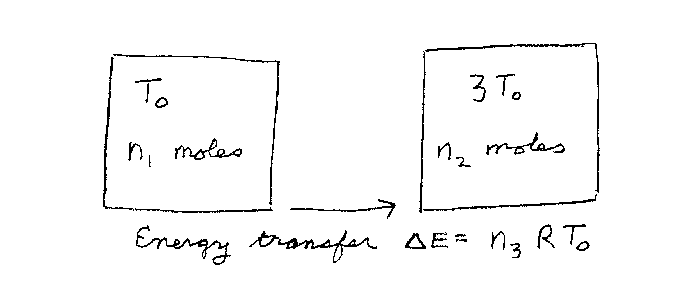Problem D6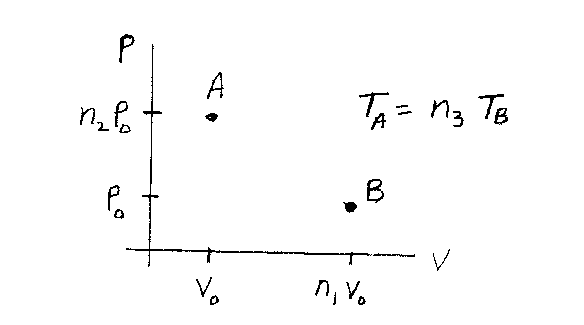Problem D7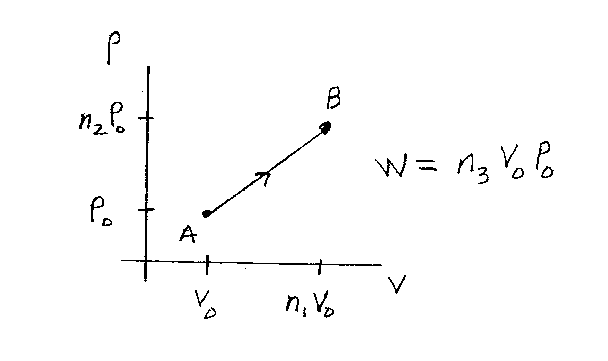Problem D8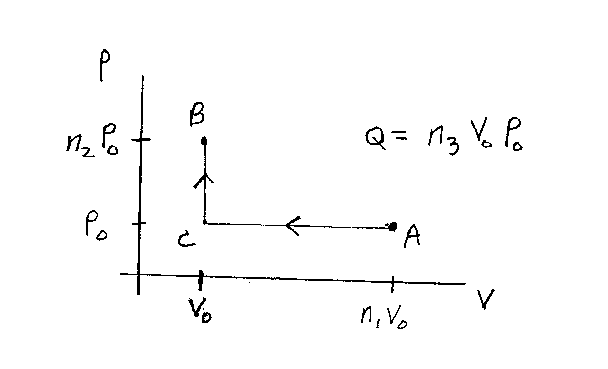Problem D9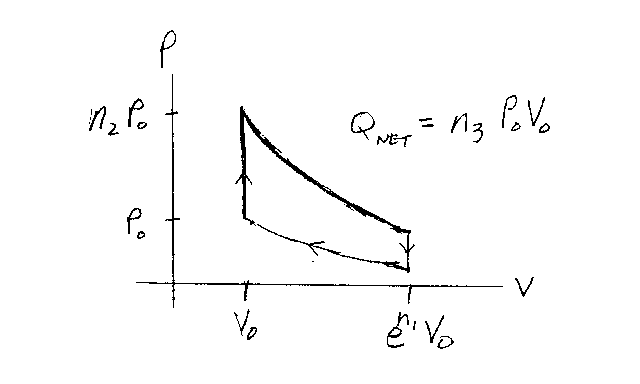Problem D10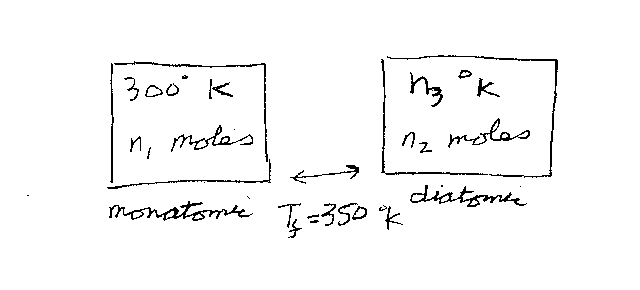Problem D11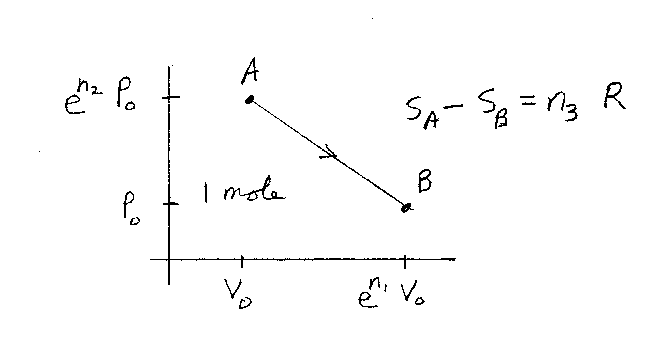Problem D12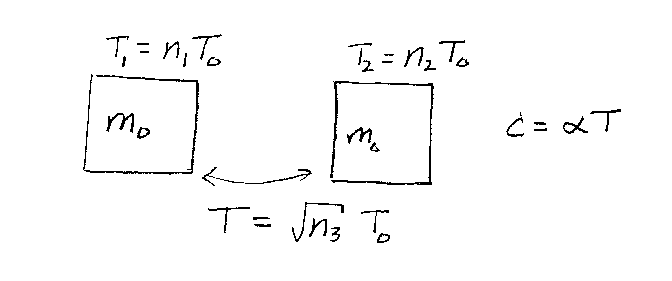Problem D13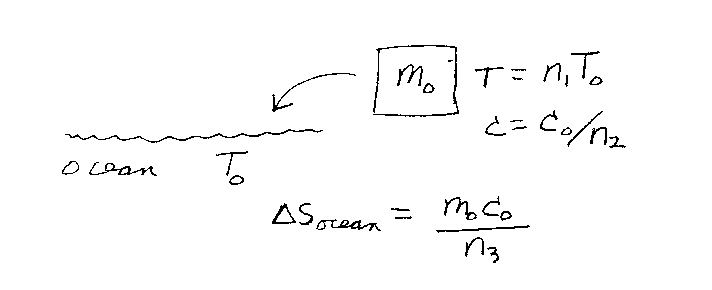Problem D14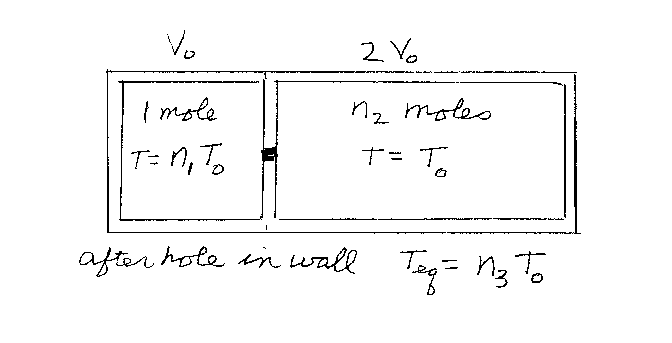Problem D15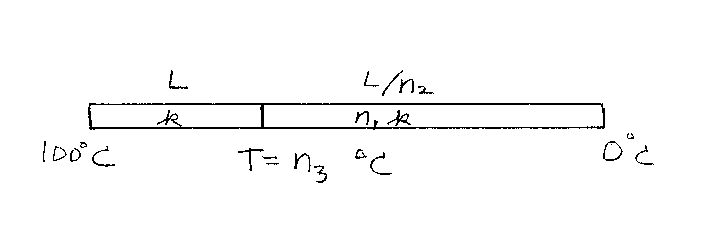Problem D16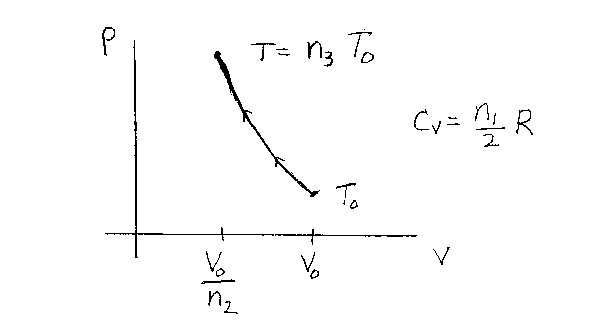Problem D17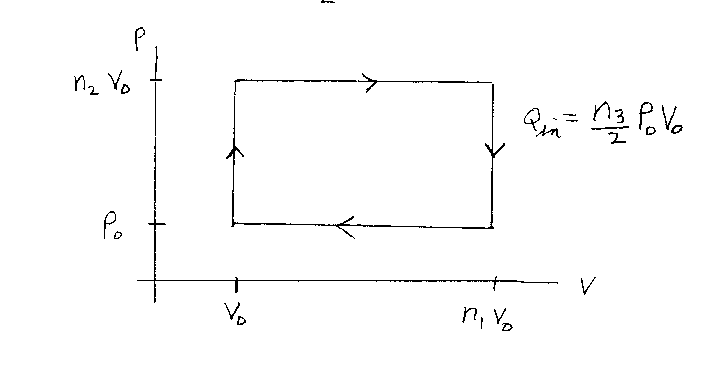Problem D18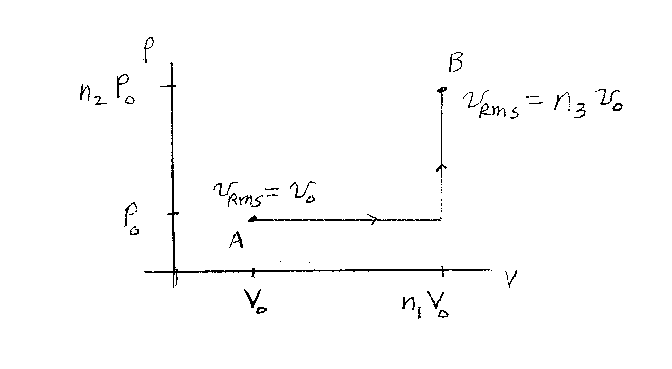Problem D19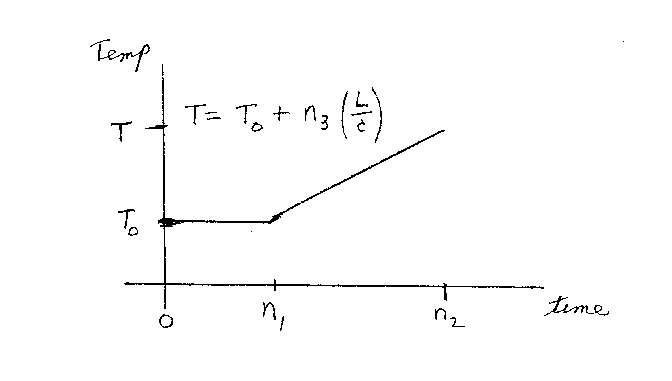Problem D20